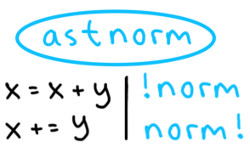# astnorm

Go AST normalization experiment.

THIS IS NOT A PROPER LIBRARY (yet?).
DO NOT USE.
It will probably be completely re-written before it becomes usable.

## Normalized code examples

1. Swap values.
Before After
```tmp := xs[i]
xs[i] = ys[i]
ys[i] = tmp
```
```xs[i], ys[i] = ys[i], xs[i]
```
1. Remove elements that are equal to `toRemove+1`.
Before After
```const toRemove = 10
var filtered []int
filtered = xs[0:0]
for i := int(0); i < len(xs); i++ {
x := xs[i]
if toRemove+1 != x {
filtered = append(filtered, x)
}
}
return (filtered)
```
```filtered := []int(nil)
filtered = xs[:0]
for _, x := range xs {
if x != 11 {
filtered = append(filtered, x)
}
}
return filtered
```

## Usage examples

Potential workflow for code searching:

### 1. Code search

• Normalize the entire Go stdlib
• Then normalize your function
• Run `grepfunc` against normalized stdlib
• If function you implemented has implementation under the stdlib, you'll probably find it

Basically, instead of stdlib you can use any kind of Go corpus.

Another code search related tasks that can be simplified by `astnorm` are code similarity evaluation and code duplication detection of any kind.

### 2. Static analysis

Suppose we have `badcode.go` file:

```package badpkg

func NotEqual(x1, x2 int) bool {
return (x1) != x1
}
```

There is an obvious mistake there, `x1` used twice, but because of extra parenthesis, linters may not detect this issue:

```\$ staticcheck badcode.go
# No output
```

Let's normalize the input first and then run `staticcheck`:

```go-normalize badcode.go > normalized_badcode.go
staticcheck normalized_badcode.go
normalized_badcode.go:4:9: identical expressions on the left and right side of the '!=' operator (SA4000)
```

And we get the warning we deserve! No changes into `staticcheck` or any other linter are required.

See also: demo script.

Get A Weekly Email With Trending Projects For These Topics
No Spam. Unsubscribe easily at any time.
go (15,168
golang (3,887
library (1,333
ast (167
types (74
source-code (55
normalization (19